# Metric system facts for kids

Kids Encyclopedia Facts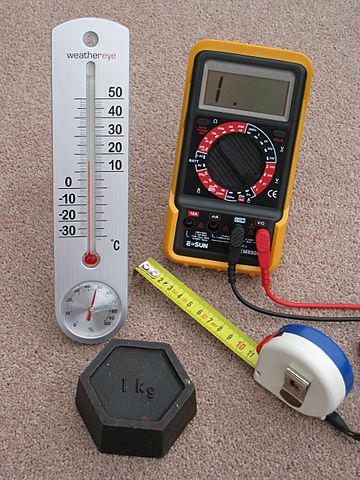A kilogram mass and three metric measuring devices: a tape measure in centimetres, a thermometer in degrees Celsius, and a multimeter that measures potential in volts, current in amperes and resistance in ohms.

The metric system is a system of measurement that succeeded the decimalised system based on the metre, which had been introduced in France in the 1790s. The historical development of these systems culminated in the definition of the International System of Units (SI) in the mid-20th century, under the oversight of an international standards body. Adopting the metric system is known as metrication.

The historical evolution of metric systems has resulted in the recognition of several principles. A set of independent dimensions of nature is selected, in terms of which all natural quantities can be expressed, called base quantities. For each of these dimensions, a representative quantity is defined as a base unit of measure. The definition of base units has increasingly been realised in terms of fundamental natural phenomena, in preference to copies of physical artefacts. A unit derived from the base units is used for expressing quantities of dimensions that can be derived from the base dimensions of the system—e.g., the square metre is the derived unit for area, which is derived from length. These derived units are coherent, which means that they involve only products of powers of the base units, without any further factors. For any given quantity whose unit has a name and symbol, an extended set of smaller and larger units is defined that are related by factors of powers of ten. The unit of time should be the second; the unit of length should be either the metre or a decimal multiple of it; and the unit of mass should be the gram or a decimal multiple of it.

Metric systems have evolved since the 1790s, as science and technology have evolved, in providing a single universal measuring system. Before and in addition to the SI, metric systems include: the MKS system of units and the MKSA systems, which are the direct forerunners of the SI; the centimetre–gram–second (CGS) system and its subtypes, the CGS electrostatic (cgs-esu) system, the CGS electromagnetic (cgs-emu) system, and their still-popular blend, the Gaussian system; the metre–tonne–second (MTS) system; and the gravitational metric systems, which can be based on either the metre or the centimetre, and either the gram, gram-force, kilogram or kilogram-force.

The SI has been adopted as the official system of weights and measures by nearly all nations in the world.

## Background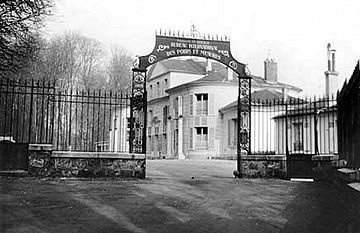Pavillon de Breteuil, Saint-Cloud, France, the home of the metric system since 1875

The French Revolution (1789–99) provided an opportunity for the French to reform their unwieldy and archaic system of many local weights and measures. Charles Maurice de Talleyrand championed a new system based on natural units, proposing to the French National Assembly in 1790 that such a system be developed. Talleyrand had ambitions that a new natural and standardised system would be embraced worldwide, and was keen to involve other countries in its development. Great Britain ignored invitations to co-operate, so the French Academy of Sciences decided in 1791 to go it alone and they set up a commission for the purpose. The commission decided that the standard of length should be based on the size of the Earth. They defined that length to be the 'metre' and its length as one ten-millionth of the length of an Earth quadrant, the length of the meridian arc on the Earth's surface from the equator to the north pole. In 1799, after the arc measurement had been surveyed, the new system was launched in France.

The units of the metric system, originally taken from observable features of nature, are now defined by seven physical constants being given exact numerical values in terms of the units. In the modern form of the International System of Units (SI), the seven base units are: metre for length, kilogram for mass, second for time, ampere for electric current, kelvin for temperature, candela for luminous intensity and mole for amount of substance. These, together with their derived units, can measure any physical quantity. Derived units may have their own unit name, such as the watt (J/s) and lux (cd/m2), or may just be expressed as combinations of base units, such as velocity (m/s) and acceleration (m/s2).

The metric system was designed to have properties that make it easy to use and widely applicable, including units based on the natural world, decimal ratios, prefixes for multiples and sub-multiples, and a structure of base and derived units. It is a coherent system, which means that its units do not introduce conversion factors not already present in equations relating quantities.

The metric system is extensible, and new derived units are defined as needed in fields such as radiology and chemistry. For example, the katal, a derived unit for catalytic activity equivalent to one mole per second (1 mol/s), was added in 1999.

## Principles

Although the metric system has changed and developed since its inception, its basic concepts have hardly changed. Designed for transnational use, it consisted of a basic set of units of measurement, now known as base units. Derived units were built up from the base units using logical rather than empirical relationships while multiples and submultiples of both base and derived units were decimal-based and identified by a standard set of prefixes.

### Realisation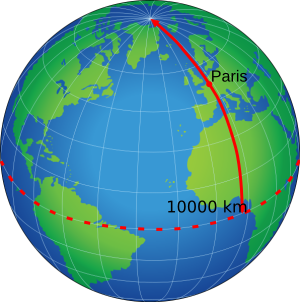The metre was originally defined to be one ten millionth of the distance between the North Pole and the Equator through Paris.

The base units used in a measurement system must be realisable. Each of the definitions of the base units in the SI is accompanied by a defined mise en pratique [practical realisation] that describes in detail at least one way in which the base unit can be measured. Where possible, definitions of the base units were developed so that any laboratory equipped with proper instruments would be able to realise a standard without reliance on an artefact held by another country. In practice, such realisation is done under the auspices of a mutual acceptance arrangement. In the SI, the standard metre is defined as exactly 1299792458 of the distance that light travels in a second. The metre can be realised by measuring the length that a light wave travels in a given time, or equivalently by measuring the wavelength of light of a known frequency.

The kilogram was originally defined as the mass of one cubic decimetre of water at 4 °C, standardized as the mass of a man-made artefact of platinum–iridium held in a laboratory in France, which was used until a new definition was introduced in May 2019. Replicas made in 1879 at the time of the artefact's fabrication and distributed to signatories of the Metre Convention serve as de facto standards of mass in those countries. Additional replicas have been fabricated since as additional countries have joined the convention. The replicas were subject to periodic validation by comparison to the original, called the IPK. It became apparent that either the IPK or the replicas or both were deteriorating, and are no longer comparable: they had diverged by 50 μg since fabrication, so figuratively, the accuracy of the kilogram was no better than 5 parts in a hundred million or a relative accuracy of 5×10−8. The accepted redefinition of SI base units replaced the IPK with an exact definition of the Planck constant as expressed in SI units, which defines the kilogram in terms of fundamental constants.

### Base and derived unit structure

A base quantity is one of a conventionally chosen subset of physical quantities, where no quantity in the subset can be expressed in terms of the others. A base unit is a unit adopted for expressing a base quantity. A derived unit is used for expressing any other quantity, and is a product of powers of base units. For example, in the modern metric system, length has the unit metre and time has the unit second, and speed has the derived unit metre per second. Density, or mass per unit volume, has the unit kilogram per cubic metre.

### Decimal ratios

A characteristic feature of metric systems is their reliance upon multiples of 10. For example, the base unit of length is the metre, and distances much longer or much shorter than 1 metre are measured in units that are powers of 10 times a metre. This is unlike older systems of units in which the ratio between the units for longer and shorter distances varied: there are 12 inches to a foot, but the number of feet in a mile (5,280) is not a power of 12. For many everyday applications, the United States has resisted the adoption of a decimal-based system, continuing to use "a conglomeration of basically incoherent measurement systems".

### Prefixes for multiples and submultiples

In the metric system, multiples and submultiples of units follow a decimal pattern.

Quick facts for kids
Metric prefixes in everyday use
Text Symbol Factor Power
yotta Y 1000000000000000000000000 1024
zetta Z 1000000000000000000000 1021
exa E 1000000000000000000 1018
peta P 1000000000000000 1015
tera T 1000000000000 1012
giga G 1000000000 109
mega M 1000000 106
kilo k 1000 103
hecto h 100 102
deca da 10 101
(none) (none) 1 100
deci d 0.1 10−1
centi c 0.01 10−2
milli m 0.001 10−3
micro μ 0.000001 10−6
nano n 0.000000001 10−9
pico p 0.000000000001 10−12
femto f 0.000000000000001 10−15
atto a 0.000000000000000001 10−18
zepto z 0.000000000000000000001 10−21
yocto y 0.000000000000000000000001 10−24

A common set of decimal-based prefixes that have the effect of multiplication or division by an integer power of ten can be applied to units that are themselves too large or too small for practical use. The concept of using consistent classical (Latin or Greek) names for the prefixes was first proposed in a report by the French Revolutionary Commission on Weights and Measures in May 1793. The prefix kilo, for example, is used to multiply the unit by 1000, and the prefix milli is to indicate a one-thousandth part of the unit. Thus the kilogram and kilometre are a thousand grams and metres respectively, and a milligram and millimetre are one thousandth of a gram and metre respectively. These relations can be written symbolically as:

1 mg = 0.001 g
1 km = 1000 m

In the early days, multipliers that were positive powers of ten were given Greek-derived prefixes such as kilo- and mega-, and those that were negative powers of ten were given Latin-derived prefixes such as centi- and milli-. However, 1935 extensions to the prefix system did not follow this convention: the prefixes nano- and micro-, for example have Greek roots. During the 19th century the prefix myria-, derived from the Greek word μύριοι (mýrioi), was used as a multiplier for 10000.

When applying prefixes to derived units of area and volume that are expressed in terms of units of length squared or cubed, the square and cube operators are applied to the unit of length including the prefix, as illustrated below.

 1 mm2 (square millimetre) = (1 mm)2 = (0.001 m)2 = 0.000001 m2 1 km2 (square kilometre) = (1 km)2 = (1000 m)2 = 1000000 m2 1 mm3 (cubic millimetre) = (1 mm)3 = (0.001 m)3 = 0.000000001 m3 1 km3 (cubic kilometre) = (1 km)3 = (1000 m)3 = 1000000000 m3

Prefixes are not usually used to indicate multiples of a second greater than 1; the non-SI units of minute, hour and day are used instead. On the other hand, prefixes are used for multiples of the non-SI unit of volume, the litre (l, L) such as millilitres (ml).

### Coherence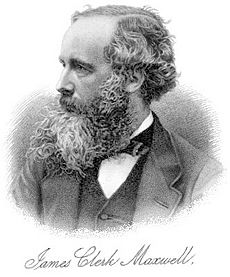James Clerk Maxwell played a major role in developing the concept of a coherent CGS system and in extending the metric system to include electrical units.

Each variant of the metric system has a degree of coherence—the derived units are directly related to the base units without the need for intermediate conversion factors. For example, in a coherent system the units of force, energy and power are chosen so that the equations

 force = mass × acceleration energy = force × distance energy = power × time

hold without the introduction of unit conversion factors. Once a set of coherent units has been defined, other relationships in physics that use this set of units will automatically be true. Therefore, Einstein's mass–energy equation, E = mc2, does not require extraneous constants when expressed in coherent units.

The CGS system had two units of energy, the erg that was related to mechanics and the calorie that was related to thermal energy; so only one of them (the erg) could bear a coherent relationship to the base units. Coherence was a design aim of SI, which resulted in only one unit of energy being defined – the joule.

### Rationalisation

Maxwell's equations of electromagnetism contained a factor of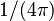$1/(4\pi)$ relating to steradians, representative of the fact that electric charges and magnetic fields may be considered to emanate from a point and propagate equally in all directions, i.e. spherically. This factor made equations more awkward than necessary, and so Oliver Heaviside suggested adjusting the system of units to remove it.

### Common notions

The basic units of the metric system, as originally defined, represented common quantities or relationships in nature. They still do – the modern precisely defined quantities are refinements of definition and methodology, but still with the same magnitudes. In cases where laboratory precision may not be required or available, or where approximations are good enough, the original definitions may suffice.

• A second is 160 of a minute, which is 160 of an hour, which is 124 of a day, so a second is 186400 of a day (the use of base 60 dates back to Babylonian times); a second is the time it takes a dense object to freely fall 4.9 metres from rest.
• The length of the equator is close to 40000000 m (more precisely 40075014.2 m). In fact, the dimensions of our planet were used by the French Academy in the original definition of the metre.
• The metre is close to the length of a pendulum that has a period of 2 seconds; most dining tabletops are about 0.75 metres high; a very tall human (basketball forward) is about 2 metres tall.
• The kilogram is the mass of a litre of cold water; a cubic centimetre or millilitre of water has a mass of one gram; a 1-euro coin weighs 7.5 g; a Sacagawea US 1-dollar coin weighs 8.1 g; a UK 50-pence coin weighs 8.0 g.
• A candela is about the luminous intensity of a moderately bright candle, or 1 candle power; a 60 W tungsten-filament incandescent light bulb has a luminous intensity of about 64 candelas.
• A mole of a substance has a mass that is its molecular mass expressed in units of grams; the mass of a mole of carbon is 12.0 g, and the mass of a mole of table salt is 58.4 g.
• Since all gases have the same volume per mole at a given temperature and pressure far from their points of liquefaction and solidification (see Perfect gas), and air is about 15 oxygen (molecular mass 32) and 45 nitrogen (molecular mass 28), the density of any near-perfect gas relative to air can be obtained to a good approximation by dividing its molecular mass by 29 (because 45 × 28 + 15 × 32 = 28.8 ≈ 29). For example, carbon monoxide (molecular mass 28) has almost the same density as air.
• A temperature difference of one kelvin is the same as one degree Celsius: 1100 of the temperature differential between the freezing and boiling points of water at sea level; the absolute temperature in kelvins is the temperature in degrees Celsius plus about 273; human body temperature is about 37 °C or 310 K.
• A 60 W incandescent light bulb rated at 120 V (US mains voltage) consumes 0.5 A at this voltage. A 60 W bulb rated at 230 V (European mains voltage) consumes 0.26 A at this voltage.

## Common metric systems

A number of different metric system have been developed, all using the Mètre des Archives and Kilogramme des Archives (or their descendants) as their base units, but differing in the definitions of the various derived units.

Variants of the metric system
Quantity SI/MKS CGS MTS
distance, length, height, etc.
(d, l, h, ...)
metre
(m)
centimetre
(cm)
metre
(m)
mass
(m)
kilogram
(kg)
gram
(g)
tonne
(t)
time
(t)
second
(s)
second
(s)
second
(s)
speed, velocity
(v, v)
m/s cm/s m/s
acceleration
(a)
m/s2 gal
(Gal)
m/s2
force
(F)
newton (N) dyne
(dyn)
sthene
(sn)
pressure
(P or p)
pascal (Pa) barye
(Ba)
pièze
(pz)
energy
(E, Q, W)
joule
(J)
erg
(erg)
kilojoule
(kJ)
power
(P)
watt
(W)
erg/s
(erg/s)
kilowatt
(kW)
viscosity
(μ)
Pa⋅s poise
(P)
pz⋅s

### 19th century

In 1832, Gauss used the astronomical second as a base unit in defining the gravitation of the earth, and together with the milligram and millimetre, this became the first system of mechanical units. He showed that the strength of a magnet could also be quantified in terms of these units, by measuring the oscillations of a magnetized needle and finding the quantity of "magnetic fluid" that produces an acceleration of one unit when applied to a unit mass. The centimetre–gram–second system of units (CGS) was the first coherent metric system, having been developed in the 1860s and promoted by Maxwell and Thomson. In 1874, this system was formally promoted by the British Association for the Advancement of Science (BAAS). The system's characteristics are that density is expressed in g/cm3, force expressed in dynes and mechanical energy in ergs. Thermal energy was defined in calories, one calorie being the energy required to raise the temperature of one gram of water from 15.5 °C to 16.5 °C. The meeting also recognised two sets of units for electrical and magnetic properties – the electrostatic set of units and the electromagnetic set of units.

The CGS units of electricity were cumbersome to work with. This was remedied at the 1893 International Electrical Congress held in Chicago by defining the "international" ampere and ohm using definitions based on the metre, kilogram and second, in the International System of Electrical and Magnetic Units. During the same period in which the CGS system was being extended to include electromagnetism, other systems were developed, distinguished by their choice of coherent base unit, including the Practical System of Electric Units, or QES (quad–eleventhgram–second) system, was being used. Here, the base units are the quad, equal to 107 m (approximately a quadrant of the earth's circumference), the eleventhgram, equal to 10−11 g, and the second. These were chosen so that the corresponding electrical units of potential difference, current and resistance had a convenient magnitude.

### 20th century

In 1901, Giovanni Giorgi showed that by adding an electrical unit as a fourth base unit, the various anomalies in electromagnetic systems could be resolved. The metre–kilogram–second–coulomb (MKSC) and metre–kilogram–second–ampere (MKSA) systems are examples of such systems.

The metre–tonne–second system of units (MTS) was based on the metre, tonne and second – the unit of force was the sthène and the unit of pressure was the pièze. It was invented in France for industrial use and from 1933 to 1955 was used both in France and in the Soviet Union. Gravitational metric systems use the kilogram-force (kilopond) as a base unit of force, with mass measured in a unit known as the hyl, Technische Masseneinheit (TME), mug or metric slug. Although the CGPM passed a resolution in 1901 defining the standard value of acceleration due to gravity to be 980.665 cm/s2, gravitational units are not part of the International System of Units (SI).

### International System of Units

The International System of Units is the modern metric system. It is based on the metre–kilogram–second–ampere (MKSA) system of units from early in the 20th century. It also includes numerous coherent derived units for common quantities like power (watt) and irradience (lumen). Electrical units were taken from the International system then in use. Other units like those for energy (joule) were modelled on those from the older CGS system, but scaled to be coherent with MKSA units. Two additional base units – the kelvin, which is equivalent to degree Celsius for change in thermodynamic temperature but set so that 0 K is absolute zero, and the candela, which is roughly equivalent to the international candle unit of illumination – were introduced. Later, another base unit, the mole, a unit of amount of substance equivalent to the Avogadro number number of specified molecules, was added along with several other derived units.

The system was promulgated by the General Conference on Weights and Measures (French: Conférence générale des poids et mesures – CGPM) in 1960. At that time, the metre was redefined in terms of the wavelength of a spectral line of the krypton-86 atom (krypton-86 being a stable isotope of an inert gas that occurs in undetectable or trace amounts naturally), and the standard metre artefact from 1889 was retired.

Today, the International system of units consists of 7 base units and innumerable coherent derived units including 22 with special names. The last new derived unit, the katal for catalytic activity, was added in 1999. All the base units except the second are now defined in terms of exact and invariant constants of physics or mathematics, barring those parts of their definitions which are dependent on the second itself. As a consequence, the speed of light has now become an exactly defined constant, and defines the metre as 1299,792,458 of the distance light travels in a second. The kilogram was defined by a cylinder of platinum-iridium alloy until a new definition in terms of natural physical constants was adopted in 2019. As of 2022, the range of decimal prefixes has been extended to those for 1030 (quetta–) and 10−30 (quecto–).In Spanish: Sistema métrico decimal para niños